# Tag: superpotential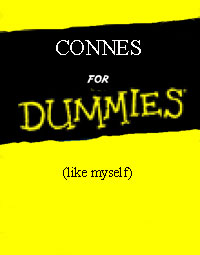You may not have noticed, but the really hard work was done behind the scenes, resurrecting about 300 old posts (some of them hidden by giving them ‘private’-status). Ive only deleted about 10 posts with little or no content and am sorry I’ve self-destructed about 20-30 hectic posts over the years by pressing the ‘delete post’ button. I would have liked to reread them after all the angry mails Ive received. But, as Ive defended myself at the time, and as I continue to do today, a blog only records feelings at a specific moment. Often, the issue is closed for me once Ive put my frustrations in a post, and then Ill forget all about it. Sadly, the gossip-circuit in noncommutative circles is a lot, a lot, slower than my mood swings, so by the time people complain it’s no longer an issue for me and I tend to delete the post altogether. A blog really is a sort of diary. For example, it only struck me now, rereading the posts of the end of 2006, beginning of 2007, how depressed I must have been at the time. Fortunately, life has improved, somewhat… Still, after all these reminiscences, the real issue is : what comes next?

Some of you may have noticed that I’ve closed the open series on tori-cryptography and on superpotentials in a rather abrupt manner. It took me that long to realize that none of you is waiting for this kind of posts. You’re thinking : if he really wants to show off, let him do his damned thing on the arXiv, a couple of days a year, at worst, and then we can then safely ignore it, like we do with most papers. Isnt’t that true? Of course it is…

So, what are you waiting for? Here’s what I believe to be a sensible thing to try out. Over the last 4 years I must have posted well over 50 times what I believe noncommutative geometry is all about, so if you still don’t know, please consult the archive, I fear I can only repeat myself. Probably, it is more worthwhile to reach out to other approaches to noncommutative geometry, trying to figure out what, if anything, they are after, without becoming a new-age convert (‘connes-vert’, I’d say). The top-left picture may give you an inkling of what I’m after… Besides, Im supposed to run a ‘capita selecta’ course for third year Bachelors and Ive chosen to read with them the book The music of the primes and to expand on the mathematics hinted only at in the book. So, I’ll totally immerse myself in Connes’ project to solve the Riemann-hypothesis in the upcoming months.

Again, rereading old posts, it strikes me how much effort I’ve put into trying to check whether technology can genuinely help mathematicians to do what they want to do more efficiently (all post categorized as iMath). I plan some series of posts re-exploring these ideas. The first series will be about the overhyped Web-2 thing of social-bookmarking. So, in the next weeks I’ll go undercover and check out which socialsites are best for mathematicians (in particular, noncommutative geometers) to embrace…

Apart from these, admittedly vague, plans I am as always open for suggestions you might have. So, please drop a comment..

It’s been a while, so let’s include a recap : a (transitive) permutation representation of the modular group $\Gamma = PSL_2(\mathbb{Z})$ is determined by the conjugacy class of a cofinite subgroup $\Lambda \subset \Gamma$, or equivalently, to a dessin d’enfant. We have introduced a quiver (aka an oriented graph) which comes from a triangulation of the compactification of $\mathbb{H} / \Lambda$ where $\mathbb{H}$ is the hyperbolic upper half-plane. This quiver is independent of the chosen embedding of the dessin in the Dedeking tessellation. (For more on these terms and constructions, please consult the series Modular subgroups and Dessins d’enfants).

Why are quivers useful? To start, any quiver $Q$ defines a noncommutative algebra, the path algebra $\mathbb{C} Q$, which has as a $\mathbb{C}$-basis all oriented paths in the quiver and multiplication is induced by concatenation of paths (when possible, or zero otherwise). Usually, it is quite hard to make actual computations in noncommutative algebras, but in the case of path algebras you can just see what happens.

Moreover, we can also see the finite dimensional representations of this algebra $\mathbb{C} Q$. Up to isomorphism they are all of the following form : at each vertex $v_i$ of the quiver one places a finite dimensional vectorspace $\mathbb{C}^{d_i}$ and any arrow in the quiver
$$\xymatrix{\vtx{v_i} \ar[r]^a & \vtx{v_j}}$$ determines a linear map between these vertex spaces, that is, to $a$ corresponds a matrix in $M_{d_j \times d_i}(\mathbb{C})$. These matrices determine how the paths of length one act on the representation, longer paths act via multiplcation of matrices along the oriented path.

A necklace in the quiver is a closed oriented path in the quiver up to cyclic permutation of the arrows making up the cycle. That is, we are free to choose the start (and end) point of the cycle. For example, in the one-cycle quiver

$$\xymatrix{\vtx{} \ar[rr]^a & & \vtx{} \ar[ld]^b \\ & \vtx{} \ar[lu]^c &}$$

the basic necklace can be represented as $abc$ or $bca$ or $cab$. How does a necklace act on a representation? Well, the matrix-multiplication of the matrices corresponding to the arrows gives a square matrix in each of the vertices in the cycle. Though the dimensions of this matrix may vary from vertex to vertex, what does not change (and hence is a property of the necklace rather than of the particular choice of cycle) is the trace of this matrix. That is, necklaces give complex-valued functions on representations of $\mathbb{C} Q$ and by a result of Artin and Procesi there are enough of them to distinguish isoclasses of (semi)simple representations! That is, linear combinations a necklaces (aka super-potentials) can be viewed, after taking traces, as complex-valued functions on all representations (similar to character-functions).

In physics, one views these functions as potentials and it then interested in the points (representations) where this function is extremal (minimal) : the vacua. Clearly, this does not make much sense in the complex-case but is relevant when we look at the real-case (where we look at skew-Hermitian matrices rather than all matrices). A motivating example (the Yang-Mills potential) is given in Example 2.3.2 of Victor Ginzburg’s paper Calabi-Yau algebras.

Let $\Phi$ be a super-potential (again, a linear combination of necklaces) then our commutative intuition tells us that extrema correspond to zeroes of all partial differentials $\frac{\partial \Phi}{\partial a}$ where $a$ runs over all coordinates (in our case, the arrows of the quiver). One can make sense of differentials of necklaces (and super-potentials) as follows : the partial differential with respect to an arrow $a$ occurring in a term of $\Phi$ is defined to be the path in the quiver one obtains by removing all 1-occurrences of $a$ in the necklaces (defining $\Phi$) and rearranging terms to get a maximal broken necklace (using the cyclic property of necklaces). An example, for the cyclic quiver above let us take as super-potential $abcabc$ (2 cyclic turns), then for example

$\frac{\partial \Phi}{\partial b} = cabca+cabca = 2 cabca$

(the first term corresponds to the first occurrence of $b$, the second to the second). Okay, but then the vacua-representations will be the representations of the quotient-algebra (which I like to call the vacualgebra)

$\mathcal{U}(Q,\Phi) = \frac{\mathbb{C} Q}{(\partial \Phi/\partial a, \forall a)}$

which in ‘physical relevant settings’ (whatever that means…) turn out to be Calabi-Yau algebras.

But, let us return to the case of subgroups of the modular group and their quivers. Do we have a natural super-potential in this case? Well yes, the quiver encoded a triangulation of the compactification of $\mathbb{H}/\Lambda$ and if we choose an orientation it turns out that all ‘black’ triangles (with respect to the Dedekind tessellation) have their arrow-sides defining a necklace, whereas for the ‘white’ triangles the reverse orientation makes the arrow-sides into a necklace. Hence, it makes sense to look at the cubic superpotential $\Phi$ being the sum over all triangle-sides-necklaces with a +1-coefficient for the black triangles and a -1-coefficient for the white ones. Let’s consider an index three example from a previous post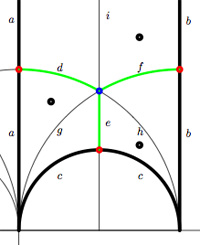$$\xymatrix{& & \rho \ar[lld]_d \ar[ld]^f \ar[rd]^e & \\ i \ar[rrd]_a & i+1 \ar[rd]^b & & \omega \ar[ld]^c \\ & & 0 \ar[uu]^h \ar@/^/[uu]^g \ar@/_/[uu]_i &}$$

In this case the super-potential coming from the triangulation is

$\Phi = -aid+agd-cge+che-bhf+bif$

and therefore we have a noncommutative algebra $\mathcal{U}(Q,\Phi)$ associated to this index 3 subgroup. Contrary to what I believed at the start of this series, the algebras one obtains in this way from dessins d’enfants are far from being Calabi-Yau (in whatever definition). For example, using a GAP-program written by Raf Bocklandt Ive checked that the growth rate of the above algebra is similar to that of $\mathbb{C}[x]$, so in this case $\mathcal{U}(Q,\Phi)$ can be viewed as a noncommutative curve (with singularities).

However, this is not the case for all such algebras. For example, the vacualgebra associated to the second index three subgroup (whose fundamental domain and quiver were depicted at the end of this post) has growth rate similar to that of $\mathbb{C} \langle x,y \rangle$…

I have an outlandish conjecture about the growth-behavior of all algebras $\mathcal{U}(Q,\Phi)$ coming from dessins d’enfants : the algebra sees what the monodromy representation of the dessin sees of the modular group (or of the third braid group).
I can make this more precise, but perhaps it is wiser to calculate one or two further examples…

Last time we have that that one can represent (the conjugacy class of) a finite index subgroup of the modular group $\Gamma = PSL_2(\mathbb{Z})$ by a Farey symbol or by a dessin or by its fundamental domain. Today we will associate a quiver to it.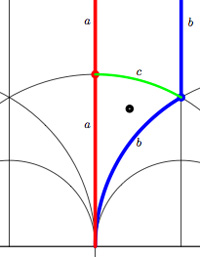For example, the modular group itself is represented by the Farey symbol
$$\xymatrix{\infty \ar@{-}[r]_{\circ} & 0 \ar@{-}[r]_{\bullet} & \infty}$$ or by its dessin (the green circle-edge) or by its fundamental domain which is the region of the upper halfplane bounded by the red and blue vertical boundaries. Both the red and blue boundary consist of TWO edges which are identified with each other and are therefore called a and b. These edges carry a natural orientation given by circling counter-clockwise along the boundary of the marked triangle (or clockwise along the boundary of the upper unmarked triangle having $\infty$ as its third vertex). That is the edge a is oriented from $i$ to $0$ (or from $i$ to $\infty$) and the edge b is oriented from $0$ to $\rho$ (or from $\infty$ to $\rho$) and the green edge c (which is an inner edge so carries no identifications) from $\rho$ to $i$. That is, the fundamental region consists of two triangles, glued together along their boundary which is the oriented cycle $\vec{abc}$ consistent with the fact that the compactification of $\mathcal{H}/\Gamma$ is the 2-sphere $S^2 = \mathbb{P}^1_{\mathbb{C}}$. Under this identification the triangle-boundary abc can be seen to circle the equator whereas the top triangle gives the upper half sphere and the lower triangle the lower half sphere. Emphasizing the orientation we can depict the triangle-boundary as the quiver

$$\xymatrix{i \ar[rd]_a & & \rho \ar[ll]_c \\ & 0 \ar[ru]_b}$$

embedded in the 2-sphere. Note that quiver is just a fancy name for an oriented graph…Okay, let’s look at the next case, that of the unique index 2 subgroup $\Gamma_2$ represented by the Farey symbol $$\xymatrix{\infty \ar@{-}[r]_{\bullet} & 0 \ar@{-}[r]_{\bullet} & \infty}$$ or the dessin (the two green edges) or by its fundamental domain consisting of the 4 triangles where again the left and right vertical boundaries are to be identified in parts.

That is we have 6 edges on the 2-sphere $\mathcal{H}/\Gamma_2 = S^2$ all of them oriented by the above rule. So, for example the lower-right triangle is oriented as $\vec{cfb}$. To see how this oriented graph (the quiver) is embedded in $S^2$ view the big lower region (cdab) as the under hemisphere and the big upper region (abcd) as the upper hemisphere. So, the two green edges together with a and b are the equator and the remaining two yellow edges form the two parts of a bigcircle connecting the north and south pole. That is, the graph are the cut-lines if we cut the sphere in 4 equal parts. The corresponding quiver-picture is

$$\xymatrix{& i \ar@/^/[dd]^f \ar@/_/[dd]_e & \\ \rho^2 \ar[ru]^d & & \rho \ar[lu]_c \\ & 0 \ar[lu]^a \ar[ru]_b &}$$As a mental check, verify that the index 3 subgroup determined by the Farey symbol $$\xymatrix{\infty \ar@{-}[r]_{\circ} & 0 \ar@{-}[r]_{\circ} & 1 \ar@{-}[r]_{\circ} & \infty}$$ , whose fundamental domain with identifications is given on the left, has as its associated quiver picture

$$\xymatrix{& & \rho \ar[lld]_d \ar[ld]^f \ar[rd]^e & \\ i \ar[rrd]_a & i+1 \ar[rd]^b & & \omega \ar[ld]^c \\ & & 0 \ar[uu]^h \ar@/^/[uu]^g \ar@/_/[uu]_i &}$$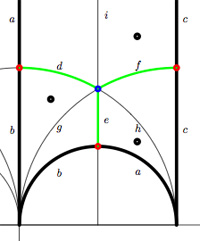whereas the index 3 subgroup determined by the Farey symbol $$\xymatrix{\infty \ar@{-}[r]_{1} & 0 \ar@{-}[r]_{1} & 1 \ar@{-}[r]_{\circ} & \infty}$$, whose fundamental domain with identifications is depicted on the right, has as its associated quiver

$$\xymatrix{i \ar[rr]^a \ar[dd]^b & & 1 \ar@/^/[ld]^h \ar@/_/[ld]_i \\ & \rho \ar@/^/[lu]^d \ar@/_/[lu]_e \ar[rd]^f & \\ 0 \ar[ru]^g & & i+1 \ar[uu]^c}$$

Next time, we will use these quivers to define superpotentials…

Here I will go over the last post at a more leisurely pace, focussing on a couple of far more trivial examples. Here’s the goal : we want to assign a quiver-superpotential to any subgroup of finite index of the modular group. So fix such a subgroup $\Gamma’$ of the modular group $\Gamma=PSL_2(\mathbb{Z})$ and consider the associated permutation representation of $\Gamma$ on the left-cosets $\Gamma/\Gamma’$. As $\Gamma \simeq C_2 \ast C_3$ this representation is determined by the action of the order 2 and order 3 generators of the modular group. There are a number of combinatorial gadgets to control the subgroup $\Gamma’$ and the associated permutation representation : (generalized) Farey symbols and dessins d’enfants.

Recall that the modular group acts on the upper-halfplane (the ‘hyperbolic plane’) by Moebius transformations, so to any subgroup $\Gamma’$ we can associate a fundamental domain for its restricted action. The dessins and the Farey symbols give us a particular choice of these fundamental domains. Let us consider the two most trivial subgroups of all : the modular group itself (so $\Gamma/\Gamma$ is just one element and therefore the associated permutation representation is just the trivial representation) and the unique index two subgroup $\Gamma_2$ (so there are two cosets $\Gamma/\Gamma_2$ and the order 2 generator interchanges these two while the order 3 generator acts trivially on them). The fundamental domains of $\Gamma$ (left) and $\Gamma_2$ (right) are depicted below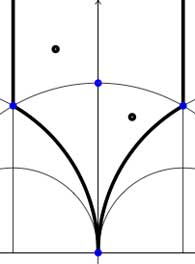In both cases the fundamental domain is bounded by the thick black (hyperbolic) edges. The left-domain consists of two hyperbolic triangles (the upper domain has $\infty$ as the third vertex) and the right-domain has 4 triangles. In general, if the subgroup $\Gamma’$ has index n, then its fundamental domain will consist of $2n$ hyperbolic triangles. Note that these triangles are part of the Dedekind tessellation so really depict the action of $PGL_2(\mathbb{Z}$ and any $\Gamma$-hyperbolic triangle consists of one black and one white triangle in Dedekind’s coloring. We will indicate the color of a triangle by a black circle if the corresponding triangle is black. Of course, the bounding edges of the fundamental domain need to be identified and the Farey symbol is a notation device to clarify this. The Farey symbols of the above domains are
$$\xymatrix{\infty \ar@{-}[r]_{\circ} & 0 \ar@{-}[r]_{\bullet} & \infty}$$ and $$\xymatrix{\infty \ar@{-}[r]_{\bullet} & 0 \ar@{-}[r]_{\bullet} & \infty}$$ respectively. In both cases this indicates that the two bounding edges on the left are to be identified as are the two bounding edges on the right (so, in particular, after identification $\infty$ coincides with $0$). Hence, after identification, the $\Gamma$ domain consists of two triangles on the vertices ${ 0,i,\rho }$ (where $\rho=e^{2 \pi i}{6}$) (the blue dots) sharing all three edges, the $\Gamma_2$ domain consists of 4 triangles on the 4 vertices ${ 0,i,\rho,\rho^2 }$ (the blue dots). In general we have three types of vertices : cusps (such as 0 or $\infty$), even vertices (such as $i$ where there are 4 hyperbolic edges in the Dedekind tessellation) and odd vertices (such as $\rho$ and $\rho^2$ where there are 6 hyperbolic edges in the tessellation).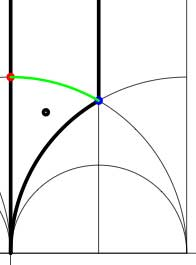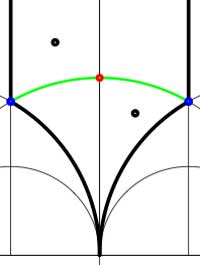Another combinatorial gadget assigned to the fundamental domain is the cuboid tree diagram or dessin. It consists of all odd and even vertices on the boundary of the domain, together with all odd and even vertices in the interior. These vertices are then connected with the hyperbolic edges connecting them. If we color the even vertices red and the odds blue we have the indicated dessins for our two examples (the green pictures). An half-edge is an edge connecting a red and a blue vertex in the dessin and we number all half-edges. So, the $\Gamma$-dessin has 1 half-edge whereas the $\Gamma_2$-dessin has two (in general, the number of these half-edges is equal to the index of the subgroup). Observe also that every triangle has exactly one half-edge as one of its three edges. The dessin gives all information to calculate the permutation representation on the coset-set $\Gamma/\Gamma’$ : the action of the order 2 generator of $\Gamma$ is given by taking for each internal red vertex the two-cycle $~(a,b)$ where a and b are the numbers of the two half-edges connected to the red vertex and the action of the order 3 generator is given by taking for every internal blue vertex the three cycle $~(c,d,e)$ where c, d and e are the numbers of the three half-edges connected to the blue vertex in counter-clockwise ordering. Our two examples above are a bit too simplistic to view this in action. There are no internal blue vertices, so the action of the order 3 generator is trivial in both cases. For $\Gamma$ there is also no red internal vertex, whence this is indeed the trivial representation whereas for $\Gamma_2$ there is one internal red vertex, so the action of the order 2 generator is given by $~(1,2)$, which is indeed the representation representation on $\Gamma/\Gamma_2$. In general, if the index of the subgroup $\Gamma’$ is n, then we call the subgroup of the symmetric group on n letters $S_n$ generated by the action-elements of the order 2 and order 3 generator the monodromy group of the permutation representation (or of the subgroup). In the trivial cases here, the monodromy groups are the trivial group (for $\Gamma$) and $C_2$ (for $\Gamma_2$).

As a safety-check let us work out all these concepts in the next simplest examples, those of some subgroups of index 3. Consider the Farey symbols

$$\xymatrix{\infty \ar@{-}[r]_{\circ} & 0 \ar@{-}[r]_{\circ} & 1 \ar@{-}[r]_{\circ} & \infty}$$ and
$$\xymatrix{\infty \ar@{-}[r]_{\circ} & 0 \ar@{-}[r]_{1} & 1 \ar@{-}[r]_{1} & \infty}$$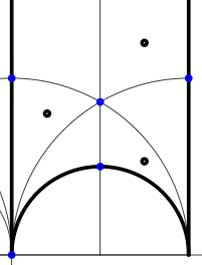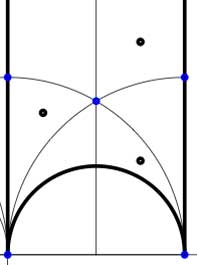In these cases the fundamental domain consists of 6 triangles with the indicated vertices (the blue dots). The distinction between the two is that in the first case, one identifies the two edges of the left, resp. bottom, resp. right boundary (so, in particular, 0,1 and $\infty$ are identified) whereas in the second one identifies the two edges of the left boundary and identifies the edges of the bottom with those of the right boundary (here, 0 is identified only with $\infty$ but also $1+i$ is indetified with $\frac{1}{2}+\frac{1}{2}i$).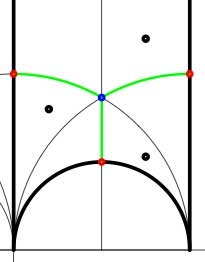In both cases the dessin seems to be the same (and given by the picture on the right). However, in the first case all three red vertices are distinct hence there are no internal red vertices in this case whereas in the second case we should identify the bottom and right-hand red vertex which then becomes an internal red vertex of the dessin!

Hence, if we order the three green half-edges 1,2,3 starting with the bottom one and counting counter-clockwise we see that in both cases the action of the order 3-generator of $\Gamma$ is given by the 3-cycle $~(1,2,3)$. The action of the order 2-generator is trivial in the first case, while given by the 2-cycle $~(1,2)$ in the second case. Therefore, the monodromy group is the cylic group $C_3$ in the first case and is the symmetric group $S_3$ in the second case.

Next time we will associate a quiver to these vertices and triangles as well as a cubic superpotential which will then allow us to define a noncommutative algebra associated to any subgroup of the modular group. The monodromy group of the situation will then reappear as a group of algebra-automorphisms of this noncommutative algebra!

Yesterday, Jan Stienstra gave a talk at theARTS entitled “Quivers, superpotentials and Dimer Models”. He started off by telling that the talk was based on a paper he put on the arXiv Hypergeometric Systems in two Variables, Quivers, Dimers and Dessins d’Enfants but that he was not going to say a thing about dessins but would rather focuss on the connection with superpotentials instead…pleasing some members of the public, while driving others to utter despair.

Anyway, it gave me the opportunity to figure out for myself what dessins might have to do with dimers, whathever these beasts are. Soon enough he put on a slide containing the definition of a dimer and from that moment on I was lost in my own thoughts… realizing that a dessin d’enfant had to be a dimer for the Dedekind tessellation of its associated Riemann surface!
and a few minutes later I could slap myself on the head for not having thought of this before :

There is a natural way to associate to a Farey symbol (aka a permutation representation of the modular group) a quiver and a superpotential (aka a necklace) defining (conjecturally) a Calabi-Yau algebra! Moreover, different embeddings of the cuboid tree diagrams in the hyperbolic plane may (again conjecturally) give rise to all sorts of arty-farty fanshi-wanshi dualities…

I’ll give here the details of the simplest example I worked out during the talk and will come back to general procedure later, when I’ve done a reference check. I don’t claim any originality here and probably all of this is contained in Stienstra’s paper or in some physics-paper, so if you know of a reference, please leave a comment. Okay, remember the Dedekind tessellation ?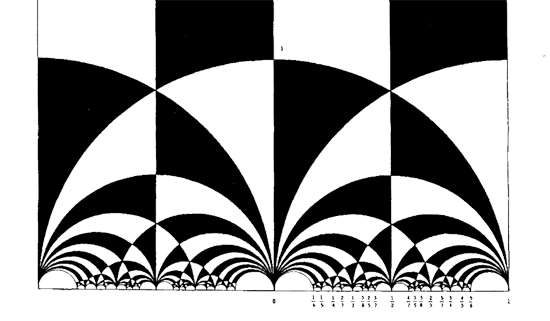So, all hyperbolic triangles we will encounter below are colored black or white. Now, take a Farey symbol and consider its associated special polygon in the hyperbolic plane. If we start with the Farey symbol

$$\xymatrix{\infty \ar@{-}_{(1)}[r] & 0 \ar@{-}_{\bullet}[r] & 1 \ar@{-}_{(1)}[r] & \infty}$$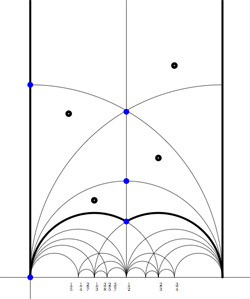we get the special polygonal region bounded by the thick edges, the vertical edges are identified as are the two bottom edges. Hence, this fundamental domain has 6 vertices (the 5 blue dots and the point at $i \infty$) and 8 hyperbolic triangles (4 colored black, indicated by a black dot, and 4 white ones).

Right, now let us associate a quiver to this triangulation (which embeds the quiver in the corresponding Riemann surface). The vertices of the triangulation are also the vertices of the quiver (so in our case we are going for a quiver with 6 vertices). Every hyperbolic edge in the triangulation gives one arrow in the quiver between the corresponding vertices. The orientation of the arrow is determined by the color of a triangle of which it is an edge : if the triangle is black, we run around its edges counter-clockwise and if the triangle is white we run over its edges clockwise (that is, the orientation of the arrow is independent of the choice of triangles to determine it). In our example, there is one arrows directed from the vertex at $i$ to the vertex at $0$, whether you use the black triangle on the left to determine the orientation or the white triangle on the right. If we do this for all edges in the triangulation we arrive at the quiver below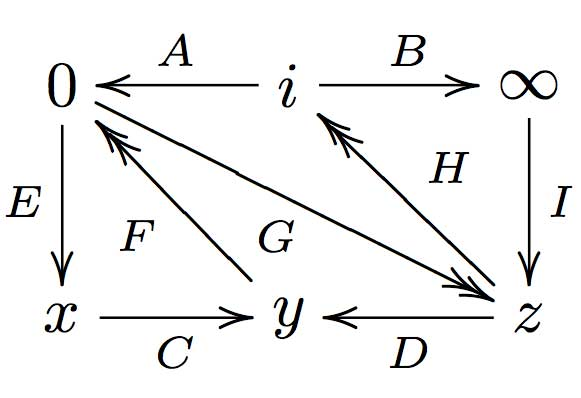where x,y and z are the three finite vertices on the $\frac{1}{2}$-axis from bottom to top and where I’ve used the physics-convention for double arrows, that is there are two F-arrows, two G-arrows and two H-arrows. Observe that the quiver is of Calabi-Yau type meaning that there are as much arrows coming into a vertex as there are arrows leaving the vertex.

Now that we have our quiver we determine the superpotential as follows. Fix an orientation on the Riemann surface (for example counter-clockwise) and sum over all black triangles the product of the edge-arrows counterclockwise MINUS sum over all white triangles
the product of the edge arrows counterclockwise. So, in our example we have the cubic superpotential

$IH’B+HAG+G’DF+FEC-BHI-H’G’A-GFD-CEF’$

From this we get the associated noncommutative algebra, which is the quotient of the path algebra of the above quiver modulo the following ‘commutativity relations’

$\begin{cases} GH &=G’H’ \\ IH’ &= IH \\ FE &= F’E \\ F’G’ &= FG \\ CF &= CF’ \\ EC &= GD \\ G’D &= EC \\ HA &= DF \\ DF’ &= H’A \\ AG &= BI \\ BI &= AG’ \end{cases}$

and morally this should be a Calabi-Yau algebra (( can someone who knows more about CYs verify this? )). This concludes the walk through of the procedure. Summarizing : to every Farey-symbol one associates a Calabi-Yau quiver and superpotential, possibly giving a Calabi-Yau algebra!